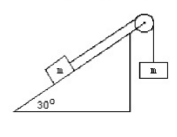# Problem: In the figure below, two masses, each 150 kg, are NOT at rest and are attached to each other by thin unstretchable cable. Find the acceleration of this system of masses and cable. The angle of the ramp is as shown in the figure. (Hint; Draw a free body diagram for each mass) Find the magnitude and direction if the coefficient of friction is 0.100.

###### Problem Details

In the figure below, two masses, each 150 kg, are NOT at rest and are attached to each other by thin unstretchable cable. Find the acceleration of this system of masses and cable. The angle of the ramp is as shown in the figure. (Hint; Draw a free body diagram for each mass) Find the magnitude and direction if the coefficient of friction is 0.100.